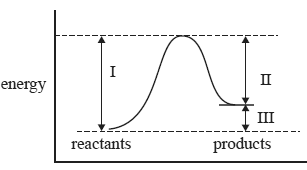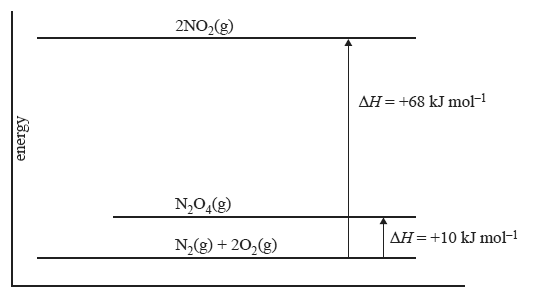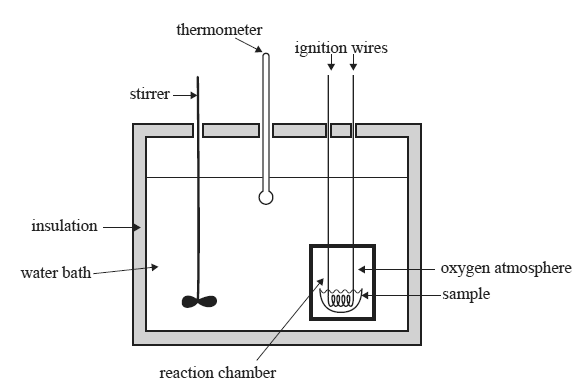Thermochemistry 2010 VCE 1) For an endothermic reaction A. the enthalpy change is negative. B. equilibrium can never be achieved. C. the reaction absorbs energy from its surroundings. D. the enthalpy of the reactants is higher than the enthalpy of the products. Solution2) For a gas phase reaction, which of the quantities (I, II and III) in the energy profile diagram above are affected by decreasing the volume of the reaction vessel at constant temperature? A. I and II only B. I and III only C. I, II and III D. none of the quantities Solution3) Magnesium metal burns in air with an intense bright light according to the equation 2 Mg(s) + O2(g) → 2 MgO(s) ΔH= –1200 kJ mol–1 How much energy is released when 7.29 g of magnesium is burned? A. 8 kJ B. 16 kJ C. 180 kJ D. 360 kJ Solution 4) The combustion of ethanol occurs according to the equation C2H5OH(1) + 3O2(g) → 2CO2 (g) + 3H2O(g) ΔH = –1364 kJ mol–1 This means that A. burning 1.0 g of liquid ethanol produces 1364 kJ of energy. B. two moles of liquid ethanol burns to produce 2728 kJ of energy. C. 1364 kJ of energy is produced when one mole of gaseous ethanol is burned. D. the activation energy for the combustion of one mole of liquid ethanol is 1364 kJ. Solution 5) The energy diagram below relates to the following two reactions. N2(g) + 2O2(g) => 2NO2(g) ΔH = +68 kJ mol–1 N2(g) + 2O2(g) =>N2O4(g) ΔH = +10 kJ mol–1The enthalpy change for the reaction NO2(g) =>1/2N2O4(g) will be A. +58 kJ mol–1 B. +29 kJ mol–1 C. –58 kJ mol–1 D. –29 kJ mol–1 Solutionb) Methane can be obtained from natural gas deposits or as a biochemical fuel from biomass. i. Which of these sources of methane would be considered more sustainable? Explain your answer. Solution ii. Energy density may be defined as the amount of energy released per gram of fuel. Use molar enthalpy of combustion data to calculate the energy density of hydrogen gas in kJ g–1. Both hydrogen and methane can be burned to produce heat energy. Solution iii. Calculate the volume of hydrogen gas in L, at SLC, that produces the same amount of energy as 2.0 L of methane gas at SLC. Solution Examiner's report for this question7) Below is a diagram of a bomb calorimeter which can be used to determine the enthalpy changes in combustion reactions. The sample is placed in the reaction chamber or 'bomb' which has a volume of 50.0 cm3. The chamber is then sealed and pure oxygen is pumped into the bomb to a pressure of 25 atm. The reaction chamber is then placed into the water bath.Solid benzoic acid, C6H5COOH, is commonly used to calibrate bomb calorimeters. 1 mol of benzoic acid releases 3227kJ of heat energy when completely combusted. a. Write a thermochemical equation for the complete combustion of benzoic acid, including a ΔH value. Solution b) A 1.025 g sample of benzoic acid was placed into the bomb. Pure oxygen was also pumped into the bomb to a pressure of 25 atm. The complete combustion of the benzoic acid created a 2.17 °C temperature rise in the surrounding water bath. The molar mass of benzoic acid is 122.0 g mol–1. i. Why must the oxygen be pumped into the bomb at high pressure? Solution ii. Calculate the calibration factor for the bomb calorimeter. Express your answer to the correct number of significant figures and include appropriate units. Solution iii. Would the value of the calibration factor be higher, lower or the same if the calibration was conducted on this calorimeter without its insulation? Explain. Solution